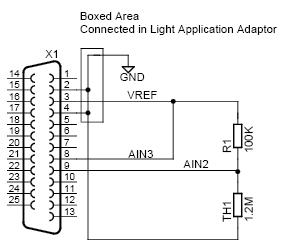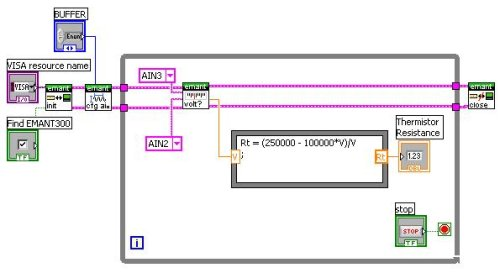The following information is for the EMANT300, EMANT380

# Measure a medical grade 1.2M @ 25c thermistor

Question: I would like to use the EMANT300 USB DAQ to measure a medical grade thermistor which has a resistance @ 25c of around 1.2M. I know that I will need to reduce the current excitation level to below 39uA, what would be the best way to do this?Answer: Connect the thermistor to the 2.5V voltage reference through a 100K 1% resistor, the voltage (V) across the 100K resistor is

V = 2.5*100000/ (Rth+100000)

where Rth is the resistance of the thermistor in ohms.

Moving the equation around gives

Rth = (250000 - 100000V)/V

LabVIEW DiagramC# code

Emant300 DAQ = new Emant300();
DAQ.Open();
DAQ.ConfigAnalog(2.5, Emant300.POLARITY.Unipolar, 100, Emant300.VREF.V2_5, true);